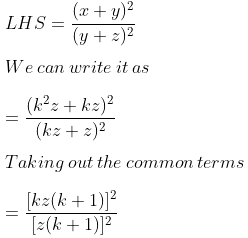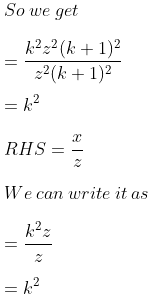Guru

# If x, y, z are in continued proportion, prove that: (x + y)2/ (y + z)2 = x/z.

• 0

exam oriented an important question from ML aggarwal, class 10th, chapter 7, ratio and proportion, Avichal publication

This question has been asked in 2010

It is given that x, y, z are in continued proportion,

prove we have to prove that: (x + y)2/ (y + z)2 = x/z.

Question no. 20, 7.2

Share

1. Solution:

It is given that

x, y, z are in continued proportion

Consider x/y = y/z = k

So we get

y = kz

x = yk = kz × k = k2zTherefore, LHS = RHS.

• 0